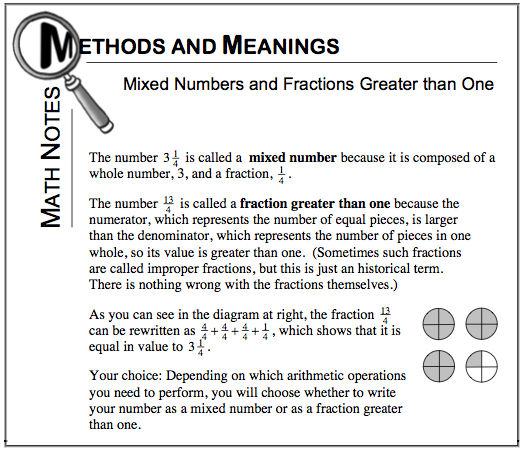### Home > MC1 > Chapter 6 > Lesson 6.3.2 > Problem6-135

6-135.
1. . Convert each mixed number to a fraction greater than one, or each fraction greater than one to a mixed number. Homework Help ✎

1. 4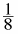2.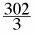3. 100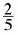4.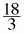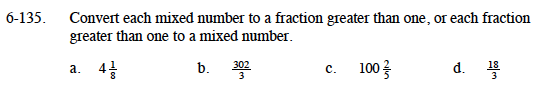Review your knowledge of mixed numbers and fractions greater than one in the Math Notes box from this lesson at the bottom of the page.

For part (a), try drawing a diagram to help visualize how many eighths are in four. Also, if you find how many eighths there are in 1, you can use multiplication to find the solution.

For part (d), you can find the mixed number by treating the fraction as a division problem. It is easier than it might seem.

$100 \frac{2}{3}$

$\frac{502}{5}$